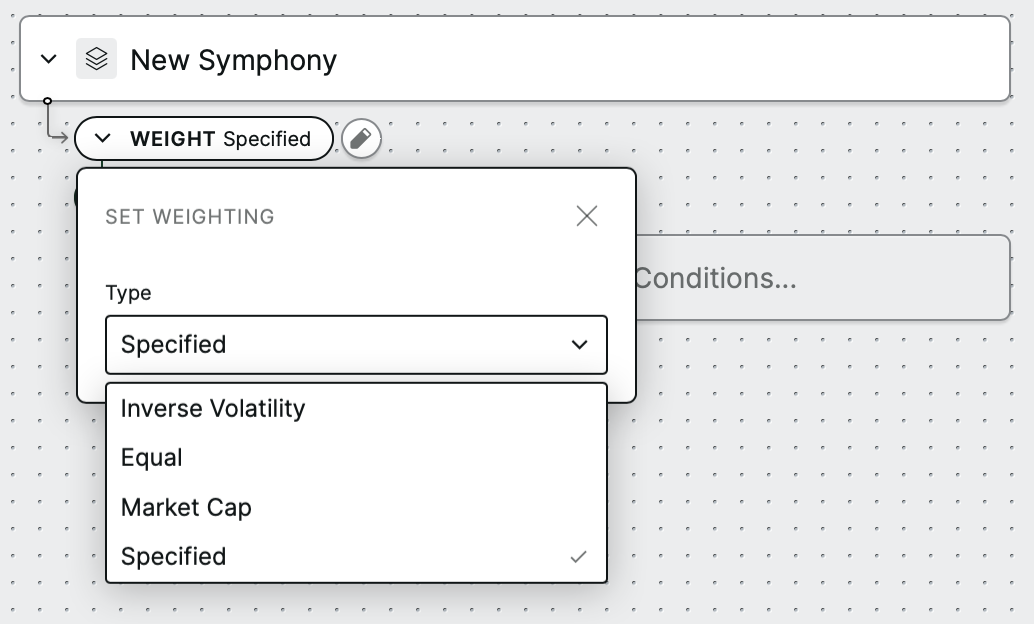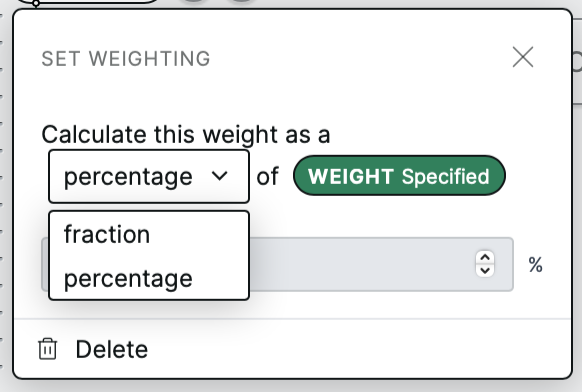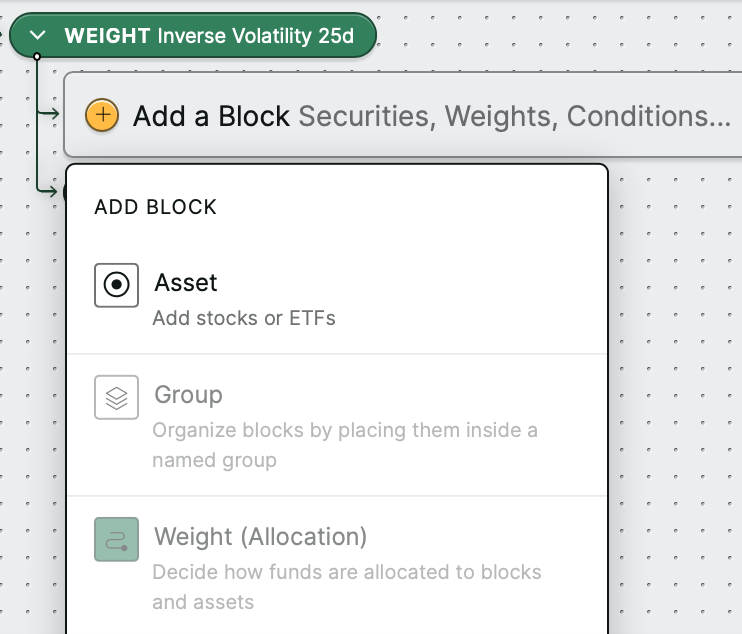# Assigning weights to blocks in a symphony

In this article, you'll learn how to assign weights to blocks in a symphony and about the types of weighting functions available in Composer.

There are currently four types of weights available: equal, specified, inverse volatility, and market cap. Equal and specified weights can be used with all types of blocks (e.g., assets, filters), while inverse volatility and market cap weightings can only be used with specific types of assets, which we'll cover below.

How do you assign a weight to a block?

Add a block under a weight block to assign it a weight.There are currently four different types of weights to choose from.Equal weighting

All of the blocks underneath an Equal weight receive an equal proportion of the weight.

For example, if there are two blocks under an Equal weight, each receives the equivalent of one half, or 50% of the weight.

Specified weighting

With specified weighting, you specify the weight of the blocks underneath this weight using a percent or fraction allocation.For example, you could assign one block 1/3 of the weight and another block 2/3 of the weight. Or, you could assign one block 40% of the weight, another block 30% of the weight, and a third block 30% of the weight.

Inverse volatility weighting

With inverse volatility weighting, assets are weighted in inverse proportion to the volatility of their returns. Assets that are more volatile receive lower weights. Selecting a lookback period is required to calculate assets' volatility.

Volatility is measured by the standard deviation (SD) of the returns of the asset over the selected lookback period. An asset's inverse volatility is 1/SD of returns. The share of weight that each asset receives is equal to its inverse volatilty divided by the total inverse volatility of all assets under the weight.

This kind of weighting can only be used with assets (stocks or ETFs), and not other kinds of blocks. If you create a new inverse volatility weight, you will only be able to add assets under it.Market cap weighting

With market cap weighting, assets are weighted by their market capitalization. The market capitalization of a company is the share price multiplied by the number of shares held by all of its shareholders. Assets with larger market capitalizations receive higher weights.

The share of weight that each asset receives is equal to its market capitalization divided by the total market capitalization of all assets under the weight.

As a result, this kind of weighting can only be used with equities (stocks), and not other kinds of assets or blocks.